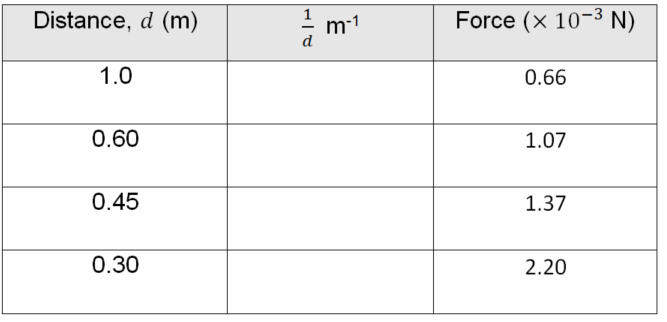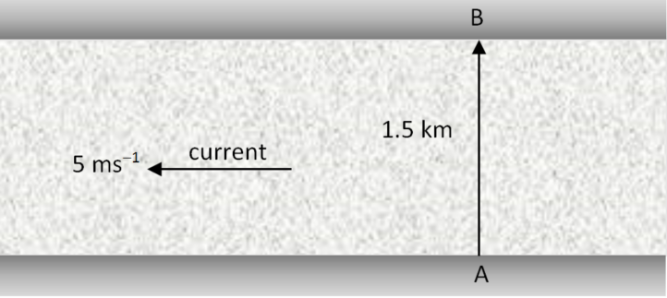# Intuition: Avoiding Easy Mistakes in Science

In this post, we explain how to use intuition to avoid making simple mistakes in science.## Would You Pay \$50 for a Cup of Coffee?

If someone charged you \$50 for a cup of coffee, you would realise something was wrong. If during a physics experiment you were holding a small piece of metal in your hand and it was labelled “50,” you would realise it probably meant 50 grams, not 50 kilograms.
In both cases, you would generally have a sense of what the answer should be in advance – because of your intuition.

Your intuition would tell you that a coffee costs a few dollars, not \$50, and that small pieces of metal which you can hold in one hand, are more likely to weigh 50 g than 50 kg. But how far does your intuition extend to the subjects you are studying and the questions you are likely to get in exams? If you develop a strong sense of intuition, you will gain an idea of whether an answer feels right – and if it doesn’t, it can trigger you to check your working for any mistakes.

## Developing Intuition

So how would you develop such a sense of intuition?

1.  Intuition comes from experience, so practising a range of questions is important.

2.  Think about whether the situation in the question is a realistic one. Your answer should make sense given the context of the question.

3. Is your answer plausible? Is there a simple qualitative comparison you can make? Is it more than something or less than something? If there is a sign or direction, does it make sense?

4. Think about the size of units of different physical quantities. For everyday situations, 1 second is a relatively short time, 1 tesla is a strong magnetic field, and 1 coulomb is a ridiculous amount of charge (if it were isolated). Being familiar with the size of units will lead to an intuition about whether the answer should be a big number or a small number.

## Where Intuition Should Detect a Mistake

In a graph question from Year 12 Physics Module 6 – Electromagnetism – students are given the data in the table below about the force between two current-carrying conductors. They are asked to complete a graph, find the gradient of the line of best fit and use it to calculate the permeability of free space μ0. Students should know that μ0 = 4π x 10-7 NA-2 = 1.26 x 10-6, so should expect an answer close to that.The force values in the table are given in units of x 10-3 N, not N. If students use the numbers given in the table and forget to account for this, their answer will be off by a factor of 1000 i.e. they will get an answer close to 1.26 x 10-3 rather than 1.26 x 10-6. For graph questions like this, intuition should tell you that being out from the expected value by 10-20% is reasonable, but being out by 1000 times is not, and it should be a trigger you to check your working.

A second example is from Y11 Physics Module 1 – Kinematics – where students are asked to work out how long it takes a boat to cross a 1.5 km wide river, given the boat can travel at 30 km/h, but the river flows at 5 ms-1. The boat must cross from A to B and is not allowed to drift downstream.This question has inconsistent units (km/h and m/s). The easiest way to answer the question is in km and hours, but many students would be used to answers being in seconds. One possible combination of mistakes can lead to an answer of 0.05 seconds. Does that answer make sense in the context of the question? No – regardless of the details, intuition should tell you that you cannot travel a distance of 1.5 km in a fraction of a second if you’re travelling at 30 km/h. The correct answer is 0.06 hours (about 3 or 4 minutes), which seems reasonable.

## Intuition in Non-intuitive Situations

We tend to have intuition about everyday situations from our everyday experiences, but what about situations we don’t experience? In Y12 Physics, relativity applies to very fast objects, and quantum physics to very small objects. We don’t have direct experience with such situations, which can lead to counter-intuitive results, like time dilation and quantisation. However, you can still develop a sense of intuition in these cases through exposure to the concepts. For example, the speed should be comparable to the speed of light for relativistic effects to be noticeable, and the effects will be stronger at higher speeds. Through practice, you can learn to consider whether an answer makes sense.

You can see from the above examples that a sense of intuition about what the answer should be, or what it should not be, can help reveal a mistake you might have made. This intuition should take into account knowledge about the size of units and the context of the question, and can be developed through practice. If your answer feels wrong, you should check it, and your intuition may save you from some silly mistakes.